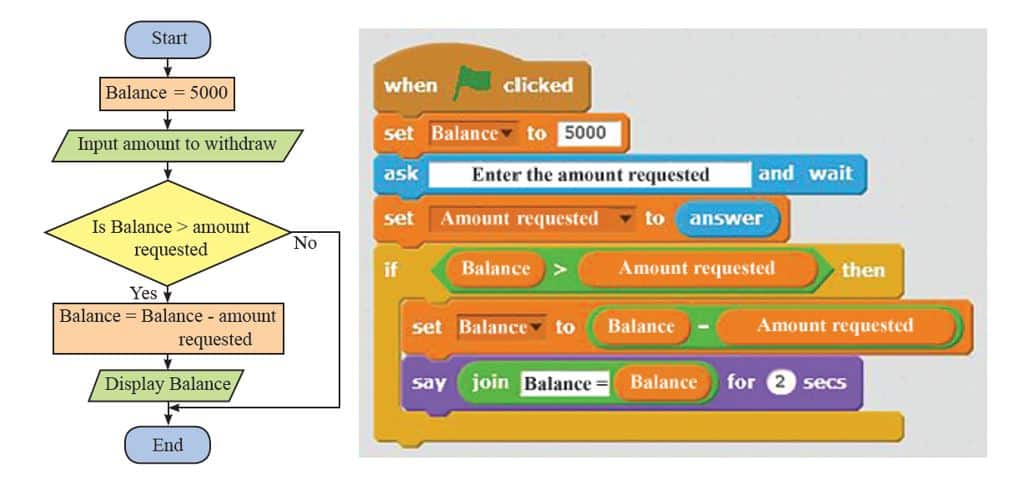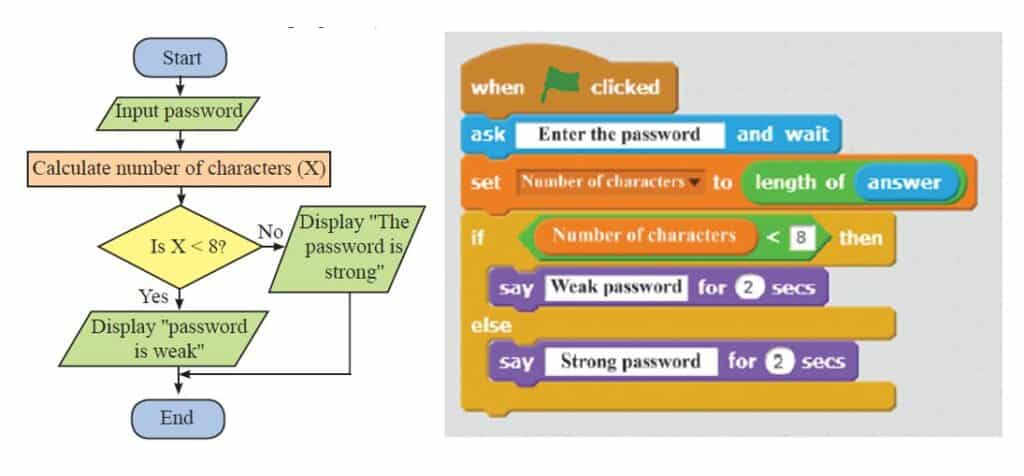# Scratch Operators Code Blocks

Scratch Operators are used to represent mathematical and logical statements in programming. We can use them to generate output by doing calculations. You can see the Operator code block in the  your Scratch software by click on the following green color circle.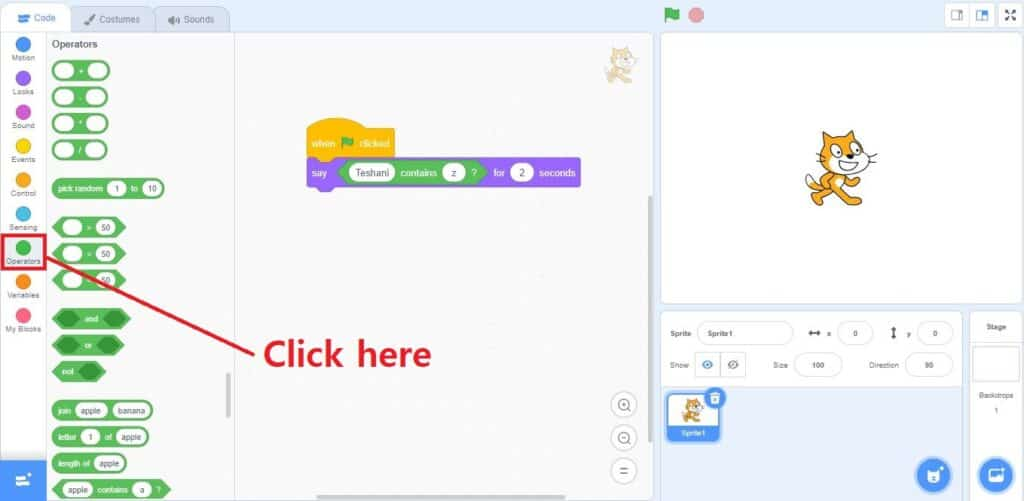Let’s learn functionality and usage of each block in Scratch Operators.

## Scratch Operators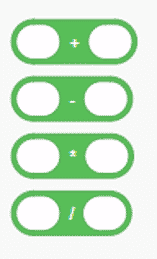These blocks use to perform arithmetical operations on the numbers and variables. You can put these blocks inside other code blocks and inside each other to create complex formula/expressions.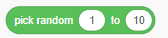This block picks a random number from a user defined range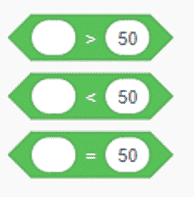These blocks test for equivalence or relative value (equal, greater than, or less than). They can be used with Control blocks to make logic tests. After doing the comparison these code blocks output either TRUE or FALSE.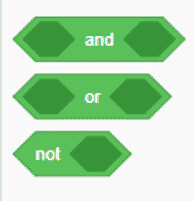These AND, OR and NOT code blocks are used for logical tests, mostly used within a control block. The output of each code block should be either TRUE or FALSE depending on the logic and it’s inputs.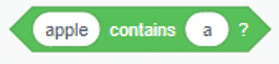This code block helps to check whether a given character exists in the given string or not. This will output TRUE  when the letter appears in the String or else FALSE.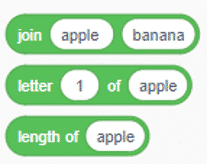These blocks are used to do things to “strings” of text, but can also be use with numbers. The JOIN operator combine two strings and output as one string. The LETTER OF function identify the character of given location. The LENGTH OF function calculates the number of characters of given string.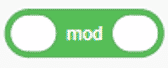The “modulo” function reports the remainder of a division operation.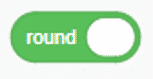The “round” function helps to round off the values by removing decimal digits.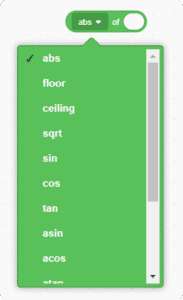This code block helps to use other mathematical operation which are required for your calculations.
Ex: Finding square root of a given number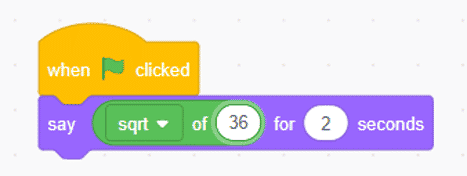### Examples to Practice Scratch Operators

Find the account balance after withdraw money